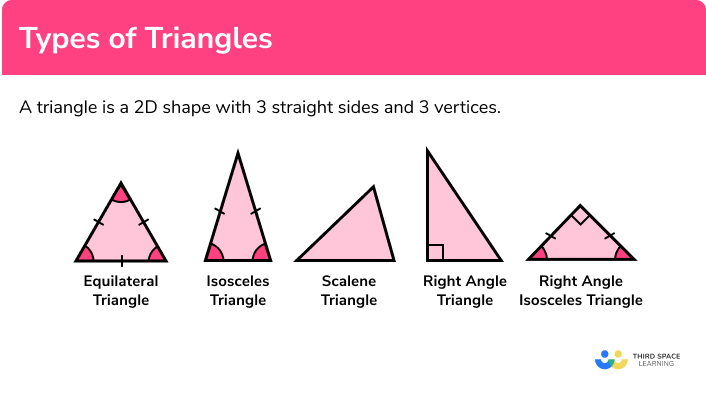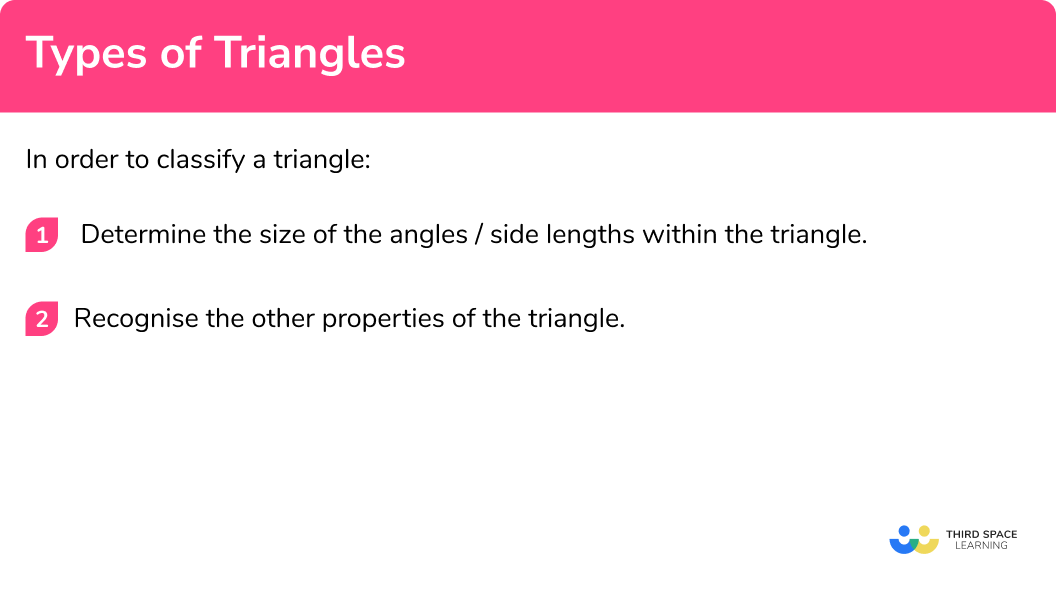# Types Of Triangles

Here we will learn about types of triangles, including their names, their properties and applying them to problems.

There are also types of triangles worksheets based on Edexcel, AQA and OCR exam questions, along with further guidance on where to go next if you’re still stuck.

## What is a triangle?

A triangle is a 2D shape with 3 straight sides and 3 vertices. You need to be able to classify triangles based on their properties, and find unknown angles and side lengths.

### What is a triangle?## Types of triangles

There are 5 different types of triangle. Below is a table that describes the properties of each triangle.

Properties of triangles are very important within geometry. They are one of the most common shapes to recognise for angles in parallel lines, circle theorems, interior angles, trigonometry, Pythagoras’ theorem, and many more. You therefore must be familiar with their individual properties.

## Triangle properties

We need to know the types of triangles and their properties. We know that all triangles have 3 sides and 3 angles. We also need to recognise that there are lots of different types of triangles and they are all unique because of the collection of properties each shape may have.

We can recognise equilateral triangles because they have 3 equal sides and 3 equal angles. Equal sides are often labelled with dashes.

E.g.

We can recognise isosceles triangles because they have 2 equal sides and 2 equal angles. The 2 equal angles are the base angles of the isosceles triangle.

E.g.

We can recognise scalene triangles because all the sides are different and all the angles are different.

E.g.

We can recognise right angled triangles because they have one right angle.

E.g.

We can recognise right angled isosceles triangles because they have 1 right angle, 2 equal sides and 2 equal angles.

E.g.

We can use these properties to solve problems and find missing angles.

E.g.

Is this statement true or false?

An equilateral triangle has three equal sized side lengths and two equal sized angles.

Equilateral triangles have 3 equal sides and 3 equal angles.

E.g.

Find the missing angle.

This is a right angled isosceles triangle because it has 1 right angle and 2 equal sides marked with a dash.

So, Angle d=45°.

## Angles in a triangle

Triangles can contain acute angles, a right angle, or one obtuse angle.

A triangle cannot contain more than one right angle, obtuse angle, or a reflex angle. The only two types of triangles that can contain an obtuse angle are an isosceles triangle and a scalene triangle.

E.g.

Here are two examples of these obtuse triangles:

The sum of angles in a triangle is 180° .

This property helps to find angles in any polygon as a polygon can be triangulated (split into triangles) and therefore the interior angles can be calculated.

Step-by-step guide: Angles in a triangle

## How to classify a triangle

In order to classify a triangle:

1. Determine the size of the angles/side lengths within the triangle.
2. Recognise the other properties of the triangle.

### How to classify a triangle### Related lessons on triangles

Types of triangles is part of our series of lessons to support revision on polygons and triangles. You may find it helpful to start with the main triangles lesson for a summary of what to expect, or use the step by step guides below for further detail on individual topics. Other lessons in this series include:

## Types of triangles examples

### Example 1: triangle

Correctly classify the following polygon:

1. Determine the size of the angles/side lengths within the triangle.

Angles in a triangle total 180° , so the missing angle is equal to:

180 - (70+55)=55° .

2Recognise the other properties of the triangle.

As two angles are the same length, the missing side must be equal to 7cm . The triangle also has one line of symmetry (vertical) and no rotational symmetry.

The polygon is an isosceles triangle.

### Example 2: multiple triangles

Two congruent, isosceles triangles are joined to form another triangle.

Use the information in the diagram below to classify the triangle ABD :

Determine the size of the angles/side lengths within the triangle.

Recognise the other properties of the polygon.

### Example 3: exterior angles

The exterior angles of a triangle are equal to 110°, 120° and 130° .

Classify the triangle.

Determine the size of the angles/side lengths within the triangle.

Recognise the other properties of the polygon.

### Example 4: algebraic

Three interior angles of a triangle are given as 2x+11, 3x+18 and x+31 .

Use this to determine the classification of the triangle.

Determine the size of the angles/side lengths within the triangle.

Recognise the other properties of the polygon.

### Example 5: description

Three people stood at different points in a field, each with a compass. The bearing of person B from person A is 120° . The bearing of person C from person B is 240° . Person C is standing directly south of person A .

If each person stood at the vertex of a triangle, what classification of triangle would they produce?

Determine the size of the angles/side lengths within the triangle.

Recognise the other properties of the polygon.

### Example 6: inscribed in a circle

The triangle ABC is inscribed in a circle.

Classify the triangle OBC .

Determine the size of the angles/side lengths within the triangle.

Recognise the other properties of the polygon.

### Common misconceptions

• Angles in polygons

Make sure you know your angle properties. Getting these confused causes quite a few misconceptions.

• Angles in a triangle total 180°.
• Angles in a quadrilateral total 360°.
• Angles on a straight line total 180°.

• Incorrect assumptions for triangles

Assuming a triangle is isosceles or equilateral can have an impact on the size of angles within the rest of the polygon, so make sure you can explain why you have chosen a specific type of triangle.

As well as this, stating that the polygon is a triangle is not enough information for a classification. You must state whether it is isosceles/ equilateral etc.

### Practice types of triangles questions

1. Classify the following triangle.Isosceles triangleScalene triangleRight angle isosceles triangleRight angle triangle180 (52 + 38) = 90^{\circ} (angles in a triangle total 180^{\circ} )

2. Use the information in the diagram to determine the type of triangle of ABD .Right angle isosceles triangleIsosceles trapeziumScalene triangleEquilateral triangleBCD = 180(110+40) = 30^{\circ} (angles in a triangle total 180^{\circ} )

ADB = 180(75 + 30 + 40) = 35^{\circ} (angles in a triangle total 180^{\circ} )

ABD = 180110 = 70^{\circ} (angles on a straight line total 180^{\circ} )

All three angles in triangle ABD are different. This is a scalene triangle.

3. The exterior angles of a triangle are equal to 120^{\circ} each. Classify the triangle.

Equilateral triangleIsosceles triangleScalene triangleRight angle isosceles triangleAs all the exterior angles are equal, the interior angles are also equal at

180 120 = 60^{\circ} .

4. Three interior angles of a triangle are given as 7x+21, 3x+12, and 2x+15 . Use this to determine the classification of the triangle.

Equilateral triangleIsosceles triangleScalene triangleRight angle triangleAs the sum of angles in a triangle total 180^{\circ} ,

7x+21+3x+12+2x+15=180\\ 12x+48=180\\ 12x=132\\ x=11

As x=11
7x+21=7(11)+21=98^{\circ}\\ 3x+12=3(11)+12=45^{\circ}\\ 2x+15=2(11)+15=37^{\circ}

5. A plane travels on a bearing of 105^{\circ} from point A , then at a bearing of 140^{\circ} from point B . The plane lands at point C . The point C is at a bearing of 137^{\circ} from point A . Classify the triangle made between points A, B, and C .

Right angle isosceles triangleScalene triangleIsosceles triangleRight angle triangleAngle BAC = 137 – 105 = 32^{\circ}

The back bearing at B = 180 – 105 = 75^{\circ}

Angle ABC = 360 – (170 + 75) = 115^{\circ} (angles at a point total 360^{\circ} )

Angle BCA = 180 – (115+32)=33^{\circ} (angles in a triangle total 180^{\circ} )

6. Use circle theorems to classify the triangle ABD .Isosceles triangleEquilateral triangleScalene triangleRight angle triangleTwo tangents that meet the circumference of a circle are the same length, so

AB = AD . This means that ABD is an isosceles triangle.

### Types of triangles GCSE questions

1.

(a) Below is a grid with three coordinates plotted.What type of triangle is ABC ?

(b) Use the grid to find the coordinate of the centre of the polygon.

(3 marks)

(a)

Isosceles triangle

(1)

(b)(1)

(3,3)

(1)

2.

(a) The rectangle ABCD and the triangle ADE are joined together as shown below. The lines AB and CE are parallel. Show that triangle ADE is a right angle isosceles triangle.(b) The line AC is joined to create a new triangle ACE with angle BAC = 42^{\circ} . Calculate the size of angle EAC . Explain your working.

(7 marks)

(a)

As AB and CE are parallel, angle ADE = 90^{\circ}

(1)

Angle DAE = 180 – (90+45) = 45^{\circ}

(1)

Angle DEA = angle DAE so the triangle is isosceles and Angle ADE is a right angle

(1)

(b)

Angles BAC and ACD are alternate angles in parallel lines which are equal

(1)

Angle ACE = 42^{\circ}

(1)

Angle EAC = 180 – (45+42) = 93^{\circ}

(1)

Angles in a triangle total 180^{\circ}

(1)

3.

(a) An isosceles triangle ABC contains the angle 50^{\circ} . Work out the possible sizes for the other two angles.

(b) A different isosceles triangle PQR is inscribed in a circle. The points P and R are connected to the centre of the circle to form a further triangle POR . What type of triangle is POR ? Explain your answer.(6 marks)

(a)

(180 -50)\div2 = 65^{\circ}

(1)

65^{\circ} and 65^{\circ}

(1)

180 (50+50) = 80^{\circ}

(1)

50^{\circ} and 80^{\circ}

(1)

(b)

Isosceles triangle

(1)

OP and OR are radii and so are the same length

(1)

## Learning checklist

You have now learned how to:

• Plot specified points and draw sides to complete a given polygon. Distinguish between regular and irregular polygons based on reasoning about equal sides and angles
• Compare and classify geometric shapes based on their properties and sizes and find unknown angles in any triangles, quadrilaterals, and regular polygons
• Describe, sketch and draw using conventional terms and notations: points, lines, parallel lines, perpendicular lines, right angles, regular polygons, and other polygons that are reflectively and rotationally symmetric
• Derive and use the sum of angles in a triangle and use it to deduce the angle sum in any polygon, and to derive properties of regular polygons

## Still stuck?

Prepare your KS4 students for maths GCSEs success with Third Space Learning. Weekly online one to one GCSE maths revision lessons delivered by expert maths tutors.

Find out more about our GCSE maths tuition programme.